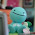## Friday, January 14, 2011

### Final Percent Post

What is a percent? A percent is out of a hundred. It is also another way of saying hundredths.

We recently learned about percents and did many things with them. We learned how to represent them in different ways, change them into fractions, decimals, and percents, how to find a percent of a number and how to combine percents.

Representing Percents:
You can use hundred grids to represent percents. You can also use more than one grid and shade part of a square.
Example: If you had to represent 180%, you would shade in one whole one hundred grid and 80 squares of another.

Fractions, Decimals, Percents:
You can use fractions and decimals to represent percents.
Example: 1/2 = 0.5 or 50%.

Percent of a Number:
To find the percent of a number, you can use some simple mental math strategies such as halving, doubling and dividing. You can also take the number and change it to a decimal then multiply that by the other number given.
Example: If you were finding 160% of \$53.27...
1.60 x 53.27 = \$85. 23

Combining Percents:
Percents can be combined by adding to help you solve problems. You can also use other strategies such as multiplying.
Example:
Ravi purchased 3 DVDs for \$19.99 each.
Find the total cost for the DVDs including
5% GST and 6% PST.

\$19.99 x 3 = \$59.97
\$59.97 x 1.11 = \$66.5667
= \$ 66.57

Here is my math video answering the four questions Mr. Harbeck gave us.

This is my percent post.
Here is a video that I hope will help you further understand percents.

1.2.